This book is archived and will be removed July 6, 2022. Please use the updated version.

The Second Law of Thermodynamics

# 25 The Carnot Cycle

### Learning Objectives

• Describe the Carnot cycle with the roles of all four processes involved
• Outline the Carnot principle and its implications
• Demonstrate the equivalence of the Carnot principle and the second law of thermodynamics

In the early 1820s, Sadi Carnot (1786−1832), a French engineer, became interested in improving the efficiencies of practical heat engines. In 1824, his studies led him to propose a hypothetical working cycle with the highest possible efficiency between the same two reservoirs, known now as the Carnot cycle. An engine operating in this cycle is called a Carnot engine. The Carnot cycle is of special importance for a variety of reasons. At a practical level, this cycle represents a reversible model for the steam power plant and the refrigerator or heat pump. Yet, it is also very important theoretically, for it plays a major role in the development of another important statement of the second law of thermodynamics. Finally, because only two reservoirs are involved in its operation, it can be used along with the second law of thermodynamics to define an absolute temperature scale that is truly independent of any substance used for temperature measurement.

With an ideal gas as the working substance, the steps of the Carnot cycle, as represented by (Figure), are as follows.

1. Isothermal expansion. The gas is placed in thermal contact with a heat reservoir at a temperature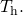The gas absorbs heat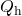from the heat reservoir and is allowed to expand isothermally, doing work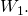Because the internal energy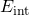of an ideal gas is a function of the temperature only, the change of the internal energy is zero, that is,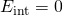during this isothermal expansion. With the first law of thermodynamics,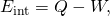we find that the heat absorbed by the gas is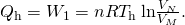The four processes of the Carnot cycle. The working substance is assumed to be an ideal gas whose thermodynamic path MNOP is represented in (Figure).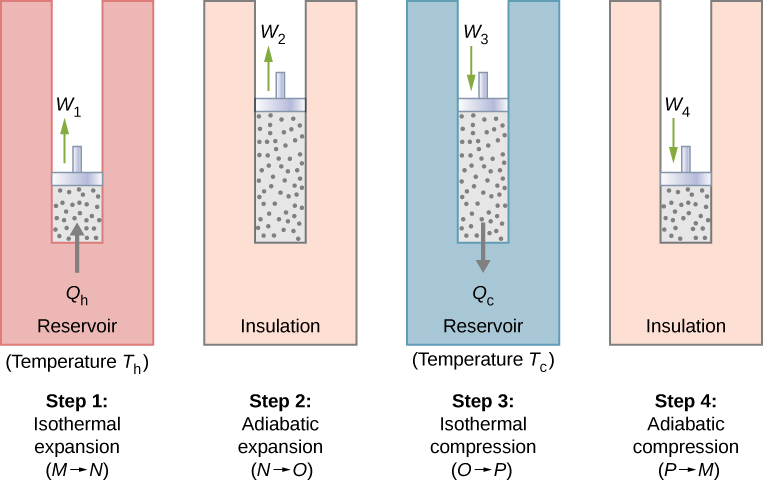The total work done by the gas in the Carnot cycle is shown and given by the area enclosed by the loop MNOPM.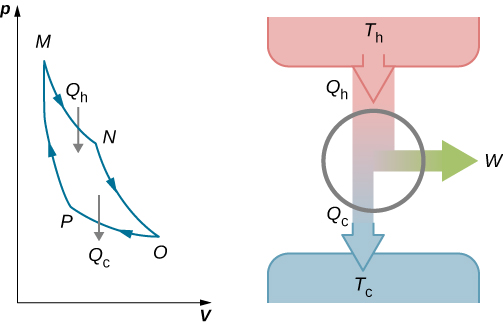2. Adiabatic expansion. The gas is thermally isolated and allowed to expand further, doing work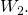Because this expansion is adiabatic, the temperature of the gas falls—in this case, from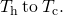From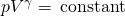and the equation of state for an ideal gas,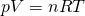, we have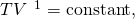so that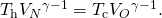3. Isothermal compression. The gas is placed in thermal contact with a cold reservoir at temperature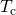and compressed isothermally. During this process, work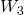is done on the gas and it gives up heat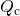to the cold reservoir. The reasoning used in step 1 now yields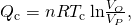whereis the heat dumped to the cold reservoir by the gas.

4. Adiabatic compression. The gas is thermally isolated and returned to its initial state by compression. In this process, work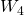is done on the gas. Because the compression is adiabatic, the temperature of the gas rises—from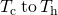in this particular case. The reasoning of step 2 now gives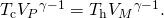The total work done by the gas in the Carnot cycle is given by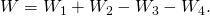This work is equal to the area enclosed by the loop shown in the pV diagram of (Figure). Because the initial and final states of the system are the same, the change of the internal energy of the gas in the cycle must be zero, that is,. The first law of thermodynamics then gives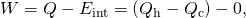and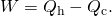To find the efficiency of this engine, we first divide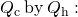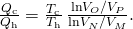When the adiabatic constant from step 2 is divided by that of step 4, we find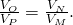Substituting this into the equation for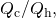we obtain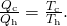Finally, with (Figure), we find that the efficiency of this ideal gas Carnot engine is given by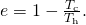An engine does not necessarily have to follow a Carnot engine cycle. All engines, however, have the same net effect, namely the absorption of heat from a hot reservoir, the production of work, and the discarding of heat to a cold reservoir. This leads us to ask: Do all reversible cycles operating between the same two reservoirs have the same efficiency? The answer to this question comes from the second law of thermodynamics discussed earlier: All reversible engine cycles produce exactly the same efficiency. Also, as you might expect, all real engines operating between two reservoirs are less efficient than reversible engines operating between the same two reservoirs. This too is a consequence of the second law of thermodynamics shown earlier.

The cycle of an ideal gas Carnot refrigerator is represented by the pV diagram of (Figure). It is a Carnot engine operating in reverse. The refrigerator extracts heatfrom a cold-temperature reservoir atwhen the ideal gas expands isothermally. The gas is then compressed adiabatically until its temperature reaches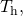after which an isothermal compression of the gas results in heatbeing discarded to a high-temperature reservoir atFinally, the cycle is completed by an adiabatic expansion of the gas, causing its temperature to drop to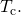The work done on the gas in one cycle of the Carnot refrigerator is shown and given by the area enclosed by the loop MPONM.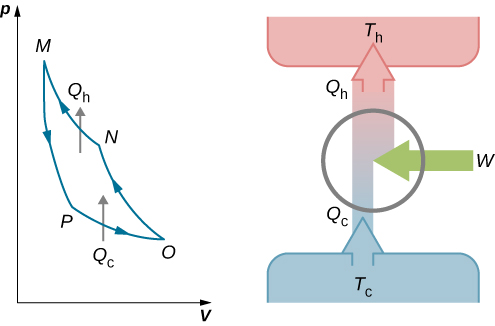The work done on the ideal gas is equal to the area enclosed by the path of the pV diagram. From the first law, this work is given byAn analysis just like the analysis done for the Carnot engine gives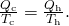When combined with (Figure), this yields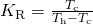for the coefficient of performance of the ideal-gas Carnot refrigerator. Similarly, we can work out the coefficient of performance for a Carnot heat pump as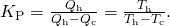We have just found equations representing the efficiency of a Carnot engine and the coefficient of performance of a Carnot refrigerator or a Carnot heat pump, assuming an ideal gas for the working substance in both devices. However, these equations are more general than their derivations imply. We will soon show that they are both valid no matter what the working substance is.

Carnot summarized his study of the Carnot engine and Carnot cycle into what is now known as Carnot’s principle:

Carnot’s Principle

No engine working between two reservoirs at constant temperatures can have a greater efficiency than a reversible engine.

This principle can be viewed as another statement of the second law of thermodynamics and can be shown to be equivalent to the Kelvin statement and the Clausius statement.

The Carnot Engine A Carnot engine has an efficiency of 0.60 and the temperature of its cold reservoir is 300 K. (a) What is the temperature of the hot reservoir? (b) If the engine does 300 J of work per cycle, how much heat is removed from the high-temperature reservoir per cycle? (c) How much heat is exhausted to the low-temperature reservoir per cycle?

Strategy From the temperature dependence of the thermal efficiency of the Carnot engine, we can find the temperature of the hot reservoir. Then, from the definition of the efficiency, we can find the heat removed when the work done by the engine is given. Finally, energy conservation will lead to how much heat must be dumped to the cold reservoir.

Solution

1. From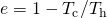we have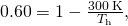so that the temperature of the hot reservoir is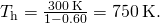2. By definition, the efficiency of the engine is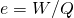, so that the heat removed from the high-temperature reservoir per cycle is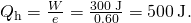3. From the first law, the heat exhausted to the low-temperature reservoir per cycle by the engine is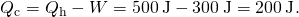Significance A Carnot engine has the maximum possible efficiency of converting heat into work between two reservoirs, but this does not necessarily mean it is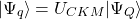efficient. As the difference in temperatures of the hot and cold reservoir increases, the efficiency of a Carnot engine increases.

A Carnot Heat Pump Imagine a Carnot heat pump operates between an outside temperature of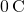and an inside temperature of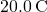. What is the work needed if the heat delivered to the inside of the house is 30.0 kJ?

Strategy Because the heat pump is assumed to be a Carnot pump, its performance coefficient is given by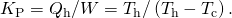Thus, we can find the work W from the heat delivered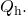Solution The work needed is obtained from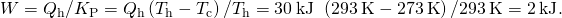Significance We note that this work depends not only on the heat delivered to the house but also on the temperatures outside and inside. The dependence on the temperature outside makes them impractical to use in areas where the temperature is much colder outside than room temperature.

In terms of energy costs, the heat pump is a very economical means for heating buildings ((Figure)). Contrast this method with turning electrical energy directly into heat with resistive heating elements. In this case, one unit of electrical energy furnishes at most only one unit of heat. Unfortunately, heat pumps have problems that do limit their usefulness. They are quite expensive to purchase compared to resistive heating elements, and, as the performance coefficient for a Carnot heat pump shows, they become less effective as the outside temperature decreases. In fact, below about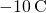, the heat they furnish is less than the energy used to operate them.

A photograph of a heat pump (large box) located outside a house. This heat pump is located in a warm climate area, like the southern United States, since it would be far too inefficient located in the northern half of the United States. (credit: modification of work by Peter Stevens)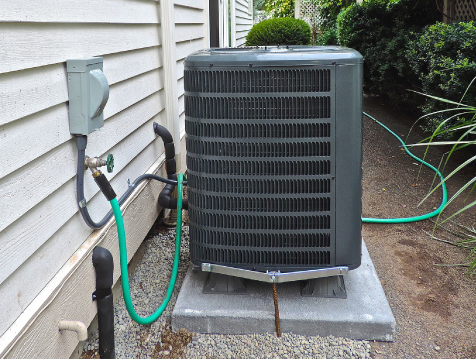Check Your Understanding A Carnot engine operates between reservoirs at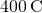and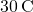. (a) What is the efficiency of the engine? (b) If the engine does 5.0 J of work per cycle, how much heat per cycle does it absorb from the high-temperature reservoir? (c) How much heat per cycle does it exhaust to the cold-temperature reservoir? (d) What temperatures at the cold reservoir would give the minimum and maximum efficiency?

a.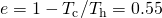; b.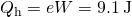; c.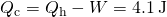; d.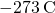and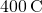Check Your Understanding A Carnot refrigerator operates between two heat reservoirs whose temperatures are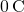and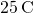. (a) What is the coefficient of performance of the refrigerator? (b) If 200 J of work are done on the working substance per cycle, how much heat per cycle is extracted from the cold reservoir? (c) How much heat per cycle is discarded to the hot reservoir?

a.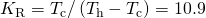; b.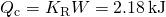; c.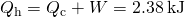### Summary

• The Carnot cycle is the most efficient engine for a reversible cycle designed between two reservoirs.
• The Carnot principle is another way of stating the second law of thermodynamics.

### Conceptual Questions

To increase the efficiency of a Carnot engine, should the temperature of the hot reservoir be raised or lowered? What about the cold reservoir?

In order to increase the efficiency, the temperature of the hot reservoir should be raised, and the cold reservoir should be lowered as much as possible. This can be seen in (Figure).

How could you design a Carnot engine withefficiency?

What type of processes occur in a Carnot cycle?

### Problems

The temperature of the cold and hot reservoirs between which a Carnot refrigerator operates are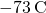and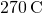, respectively. Which is its coefficient of performance?

2.0

Suppose a Carnot refrigerator operates between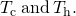Calculate the amount of work required to extract 1.0 J of heat from the cold reservoir if (a)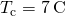,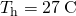; (b)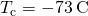,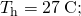(c)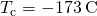,; and (d)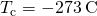,.

A Carnot engine operates between reservoirs at 600 and 300 K. If the engine absorbs 100 J per cycle at the hot reservoir, what is its work output per cycle?

50 J

A 500-W motor operates a Carnot refrigerator between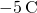and. (a) What is the amount of heat per second extracted from the inside of the refrigerator? (b) How much heat is exhausted to the outside air per second?

Sketch a Carnot cycle on a temperature-volume diagram.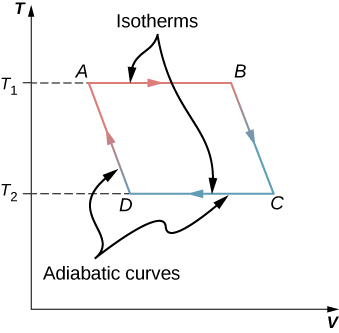A Carnot heat pump operates betweenand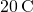. How much heat is exhausted into the interior of a house for every 1.0 J of work done by the pump?

An engine operating between heat reservoirs at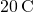and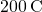extracts 1000 J per cycle from the hot reservoir. (a) What is the maximum possible work that engine can do per cycle? (b) For this maximum work, how much heat is exhausted to the cold reservoir per cycle?

a. 381 J; b. 619 J

Suppose a Carnot engine can be operated between two reservoirs as either a heat engine or a refrigerator. How is the coefficient of performance of the refrigerator related to the efficiency of the heat engine?

A Carnot engine is used to measure the temperature of a heat reservoir. The engine operates between the heat reservoir and a reservoir consisting of water at its triple point. (a) If 400 J per cycle are removed from the heat reservoir while 200 J per cycle are deposited in the triple-point reservoir, what is the temperature of the heat reservoir? (b) If 400 J per cycle are removed from the triple-point reservoir while 200 J per cycle are deposited in the heat reservoir, what is the temperature of the heat reservoir?

a. 546 K; b. 137 K

What is the minimum work required of a refrigerator if it is to extract 50 J per cycle from the inside of a freezer atand exhaust heat to the air at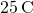?

### Glossary

Carnot cycle
cycle that consists of two isotherms at the temperatures of two reservoirs and two adiabatic processes connecting the isotherms
Carnot engine
Carnot heat engine, refrigerator, or heat pump that operates on a Carnot cycle
Carnot principle
principle governing the efficiency or performance of a heat device operating on a Carnot cycle: any reversible heat device working between two reservoirs must have the same efficiency or performance coefficient, greater than that of an irreversible heat device operating between the same two reservoirs